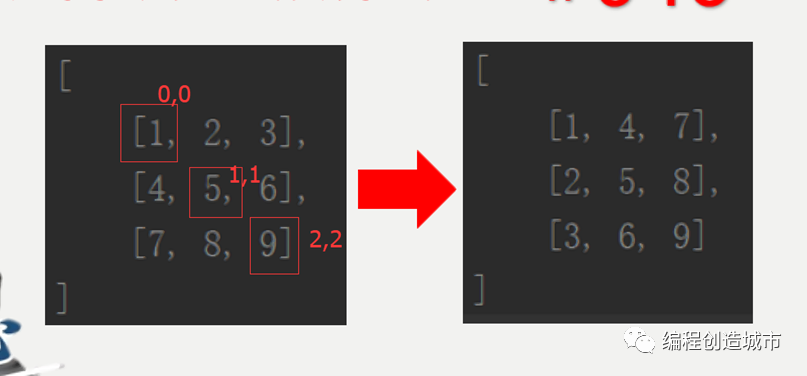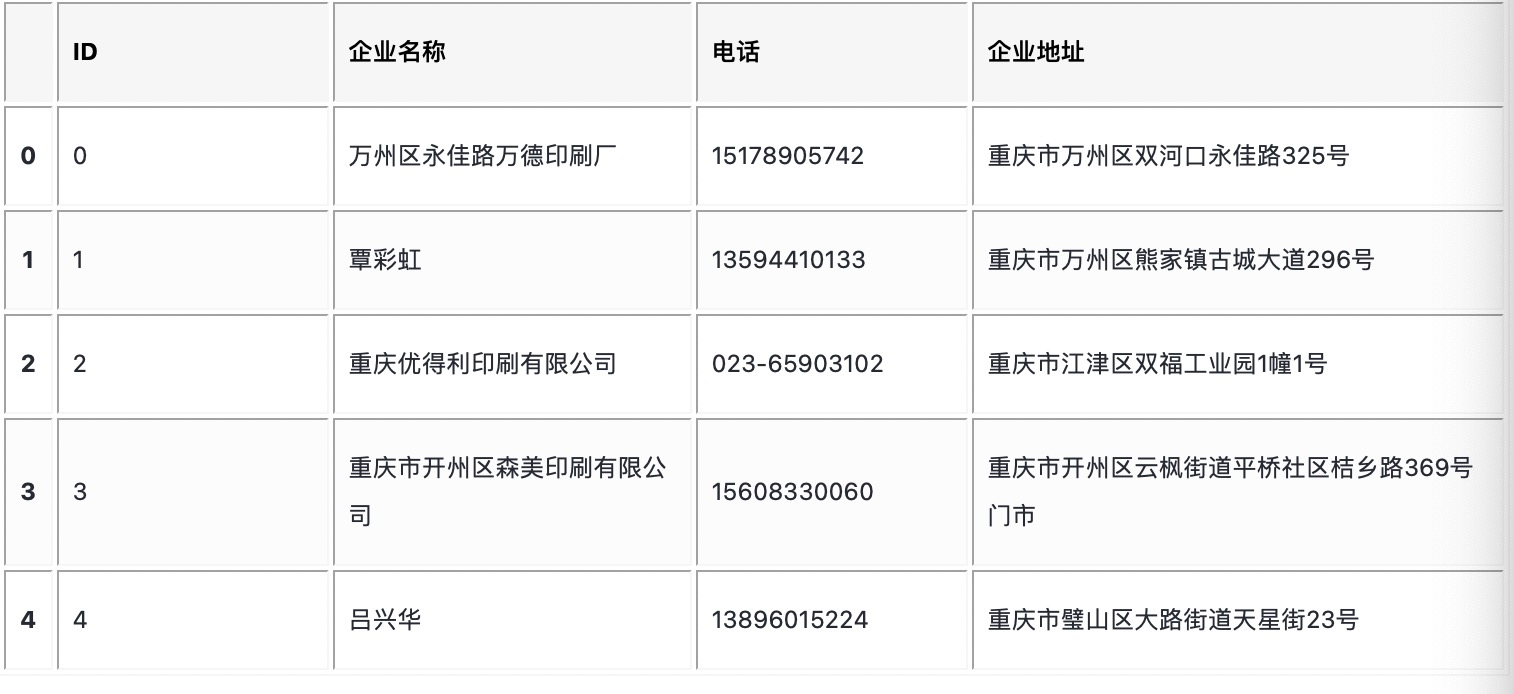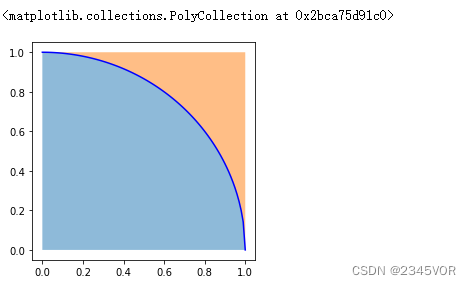+关注继续查看

# Python机器学习：通过scikit-learn实现集成算法

scikit-learn是Python中开发和实践机器学习的著名类库之一，依赖于SciPy及其相关类库来运行。scikit-learn的基本功能主要分为六大部分：分类、回归、聚类、数据降维、模型选择和数据预处理。需要指出的是，由于scikit-learn本身不支持深度学习，也不支持GPU加速，因此scikit-learn对于多层感知器（MLP）神经网络的实现并不适合处理大规模问题。（scikit-learn对MLP的支持在0.18版之后增加）

scikit-learn是一个开源项目，遵守BSD协议，可以将项目应用于商业开发。目前主要由社区成员自发进行维护。可能是由于维护成本的限制，scikit-learn相比其他项目要显得更为保守，这主要体现在两个方面：

scikit-learn从来不做除机器学习领域之外的其他扩展。

scikit-learn从来不采用未经广泛验证的算法。

## 2 装袋算法

### 2.1 装袋决策树

from pandas import read_csv
from sklearn.model_selection import KFold
from sklearn.model_selection import cross_val_score
from sklearn.ensemble import BaggingClassifier
from sklearn.tree import DecisionTreeClassifier
# 导入数据
filename = 'pima_data.csv'
names = ['preg', 'plas', 'pres', 'skin', 'test', 'mass', 'pedi', 'age', 'class']
# 将数据分为输入数据和输出结果
array = data.values
X = array[:, 0:8]
Y = array[:, 8]
num_folds = 10
seed = 7
kfold = KFold(n_splits=num_folds, random_state=seed)
cart = DecisionTreeClassifier()
num_tree = 100
model = BaggingClassifier(base_estimator=cart, n_estimators=num_tree, random_state=seed)
result = cross_val_score(model, X, Y, cv=kfold)
print(result.mean())


0.770745044429


### 2.2 随机森林

from pandas import read_csv
from sklearn.model_selection import KFold
from sklearn.model_selection import cross_val_score
from sklearn.ensemble import RandomForestClassifier
# 导入数据
filename = 'pima_data.csv'
names = ['preg', 'plas', 'pres', 'skin', 'test', 'mass', 'pedi', 'age', 'class']
# 将数据分为输入数据和输出结果
array = data.values
X = array[:, 0:8]
Y = array[:, 8]
num_folds = 10
seed = 7
kfold = KFold(n_splits=num_folds, random_state=seed)
num_tree = 100
max_features = 3
model = RandomForestClassifier(n_estimators=num_tree, random_state=seed, max_features=max_features)
result = cross_val_score(model, X, Y, cv=kfold)
print(result.mean())


0.77337662337


### 2.3 极端随机树

（1）随机森林应用的是Bagging模型，而极端随机树是使用所有的训练样本得到每棵决策树，也就是每棵决策树应用的是相同的全部训练样本。

（2）随机森林是在一个随机子集内得到最优分叉特征属性，而极端随机树是完全随机地选择分叉特征属性，从而实现对决策树进行分叉的。

from pandas import read_csv
from sklearn.model_selection import KFold
from sklearn.model_selection import cross_val_score
from sklearn.ensemble import ExtraTreesClassifier
# 导入数据
filename = 'pima_data.csv'
names = ['preg', 'plas', 'pres', 'skin', 'test', 'mass', 'pedi', 'age', 'class']
# 将数据分为输入数据和输出结果
array = data.values
X = array[:, 0:8]
Y = array[:, 8]
num_folds = 10
seed = 7
kfold = KFold(n_splits=num_folds, random_state=seed)
num_tree = 100
max_features = 7
model = ExtraTreesClassifier(n_estimators=num_tree, random_state=seed, max_features=max_features)
result = cross_val_score(model, X, Y, cv=kfold)
print(result.mean())


0.762987012987

## 3 提升算法

from pandas import read_csv
from sklearn.model_selection import KFold
from sklearn.model_selection import cross_val_score
# 导入数据
filename = 'pima_data.csv'
names = ['preg', 'plas', 'pres', 'skin', 'test', 'mass', 'pedi', 'age', 'class']
# 将数据分为输入数据和输出结果
array = data.values
X = array[:, 0:8]
Y = array[:, 8]
num_folds = 10
seed = 7
kfold = KFold(n_splits=num_folds, random_state=seed)
num_tree = 30
result = cross_val_score(model, X, Y, cv=kfold)
print(result.mean())


0.76045796309

### 3.2 随机梯度提升

from pandas import read_csv
from sklearn.model_selection import KFold
from sklearn.model_selection import cross_val_score
# 导入数据
filename = 'pima_data.csv'
names = ['preg', 'plas', 'pres', 'skin', 'test', 'mass', 'pedi', 'age', 'class']
# 将数据分为输入数据和输出结果
array = data.values
X = array[:, 0:8]
Y = array[:, 8]
num_folds = 10
seed = 7
kfold = KFold(n_splits=num_folds, random_state=seed)
num_tree = 100
result = cross_val_score(model, X, Y, cv=kfold)
print(result.mean())


0.766900205058


## 4 投票算法

from pandas import read_csv
from sklearn.model_selection import KFold
from sklearn.model_selection import cross_val_score
from sklearn.ensemble import VotingClassifier
from sklearn.tree import DecisionTreeClassifier
from sklearn.svm import SVC
from sklearn.linear_model import LogisticRegression
# 导入数据
filename = 'pima_data.csv'
names = ['preg', 'plas', 'pres', 'skin', 'test', 'mass', 'pedi', 'age', 'class']
# 将数据分为输入数据和输出结果
array = data.values
X = array[:, 0:8]
Y = array[:, 8]
num_folds = 10
seed = 7
kfold = KFold(n_splits=num_folds, random_state=seed)
cart = DecisionTreeClassifier()
models = []
model_logistic = LogisticRegression()
models.append(('logistic', model_logistic))
model_cart = DecisionTreeClassifier()
models.append(('cart', model_cart))
model_svc = SVC()
models.append(('svm', model_svc))
ensemble_model = VotingClassifier(estimators=models)
result = cross_val_score(ensemble_model, X, Y, cv=kfold)
print(result.mean())


0.73299726589228 019 0Python 源代码里的算法——如何合并多个有序列表并使得结果依然有序？
Python 源代码里的算法——如何合并多个有序列表并使得结果依然有序？
12 029 0python散列表实现查找，使用了多种算法并测试对比进行了性能分析(查找效率)

22 0HaaS Python 2.1 版本更新上线 在线热更新+智能行为感知算法+更多开发板/API+精彩开发者活动 快来体验
HaaS Python 2.1 版本更新上线 在线热更新+智能行为感知算法+更多开发板/API+精彩开发者活动 快来体验
21 02023java面试算法真题 python go rust js 解法
2023java面试算法真题 python go rust js 解法
37 0Python缓存神器cachetools：提高程序性能的利器，一文详解其缓存算法
Python缓存神器cachetools：提高程序性能的利器，一文详解其缓存算法
4996 0【Python蒙特卡罗算法】
【Python蒙特卡罗算法】
69 0

【Python入门】Python数据处理编程基础3416